blog

# Predicting and Reducing Energy Consumption of Machine Learning Models

In this blog post, we will explore ways to predict the energy consumption of Machine Learning models based on the number of CPU operations they require (FLOPs). We then look at some of the factors that may also need to be considered to develop accurate and reliable predictions.

## Climate Change and AI

Recent studies have found that the energy cost of training one large Natural Language Processing model is significant and training one single transformer model is estimated to generate approximately the same lifetime CO₂ emissions as five average cars.

To develop AI and Machine Learning models responsibly, we ought to derive tools for the AI community to lower its greenhouse gas emissions and energy consumption.

## Green AI and the AI Community

Green AI is an approach to yielding State-Of-The-Art (SOTA) results while consuming less energy than existing solutions. The term was developed by Schwartz et al. to try to change how the AI community thinks about AI development, suggesting that the AI community needs to construct AI systems that deliver a more sustainable world.

When we talk about an AI system, the AI community often divides it into two main phases: training and inference. Researchers have studied the training phase of many AI systems’ life cycles, and methods of enabling Green AI have been established. However, less research has been conducted regarding inference.

Accurately predicting energy consumption requires the right metrics. However, currently, metrics of this sort are not standardised, and the closest metric to experiment with is Floating Point Operations (FLOPs). To predict the energy consumption of future AI work, we need to understand how FLOPs can affect different machines and models.

## Introducing FLOPs

If we take a step back and think about what is needed to predict any cost, we first need to know what work we want to do, so that we can analyse the operating expenditures required for us to perform that work.

There are no standardised metrics today, but Henderson et al. took the first step with their paper“Towards the Systematic Reporting of the Energy and Carbon Footprints of Machine Learning’’. The findings all pointed to the same metric: FLOPs.

However, FLOPs as a metric are not sufficiently informative for us to know how much work will be performed. We also need to consider our inference machine, and how quickly we want the work to be done.

For this, we can use Floating-Point Operations per Second (FLOPS, note the capital S here). This metric is calculated using the following equation: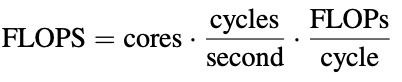Fig 1: The equation for FLOPS

Efficiency is defined as the number of FLOPS per Watt: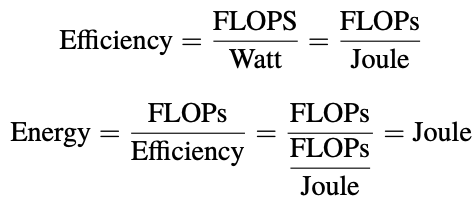Fig 2: The equations for Efficiency and Energy

We also need to consider what kind of FLOPs are handled. A machine has multiple components that use FLOPs of various forms and other parts that do not handle FLOPs, such as memory. Let’s look at an example of GPU Efficiency (TFLOPs per joule) of multiple precisions. We’ll compare the Nvidia A100 to the new H100 released this year (2022).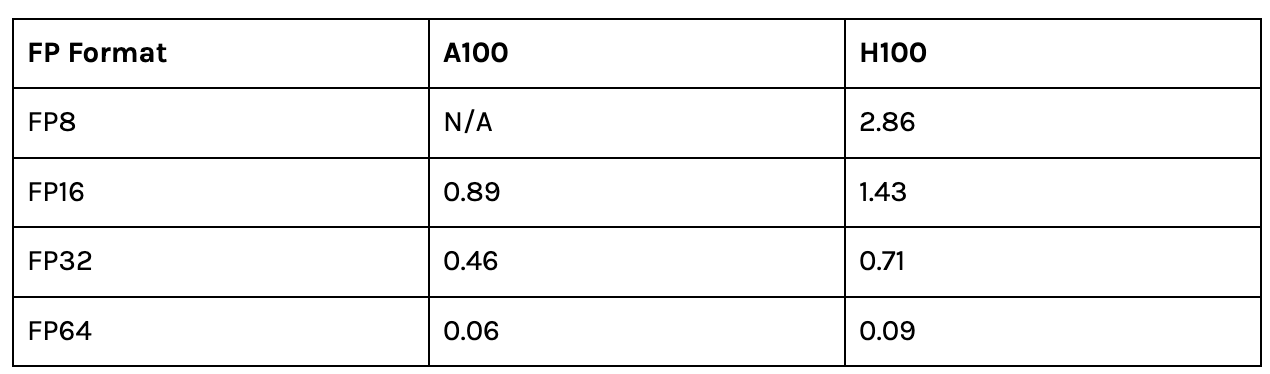Fig 3: GPU Efficiency (TFLOPs per joule).

Although the H100’s TDP is 700W compared to the A100’s 350W, the H100 uses its watts more efficiently than the A100. For Floating-Point (FP) precision of 32-bit, the H100 performs 250,000,000,000 more floating-point operations than the A100 per joule.

We cannot use efficiency in the same way for memory; memory does not execute an instruction as the CPU or GPU does. Instead, the memory ensures that data is available and accessible. To accurately estimate the memory energy consumption, we would need to estimate the number of memory operations it can perform during inference.

Going forward, we now define some limitations before looking at the empirical research.

• We will only examine 32-bit Floating-Point (FP) precision
• We will include the memory energy consumption as a part of the CPU to predict memory energy together with CPU energy (GPU integrated memory)
• We estimate the fine-tuning process to save time and energy, meaning we measure energy consumption for x steps and then multiply it to get all steps in the y epochs.

Here are the models used for data collection: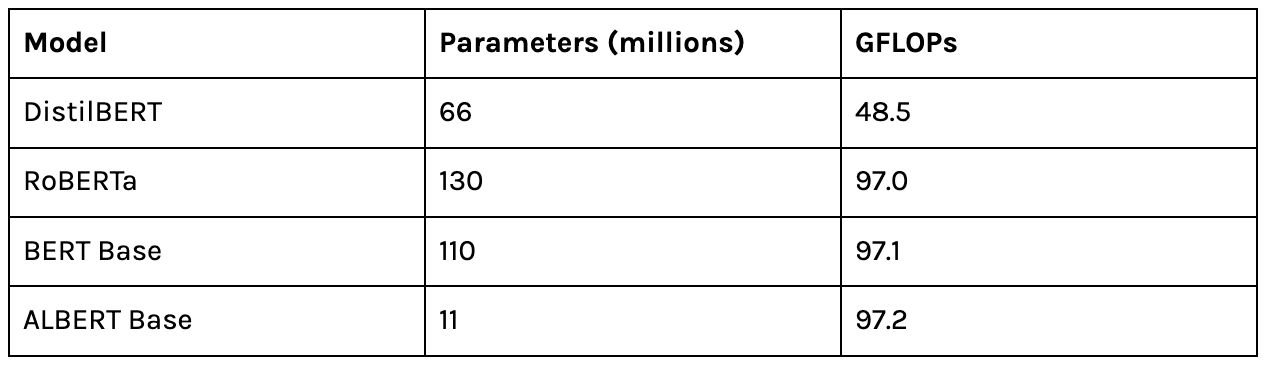Figure 4: Models used in data collection

## Floating-Point Operations and Energy Predictions

To predict model energy consumption, we divide the cost source into different phases seen below.

• Fine-tuning: Training and retraining the model
• Cold-start: Starting up the Inference Server
• Inference: The model works with requests

Fine-tuning

In Figure 1, we see each model with a null value for no work done. The fitted line reveals a strong correlation with R² = 0.952, which indicates that FLOPs are a good predictor of energy consumption regarding the CPU and DRAM.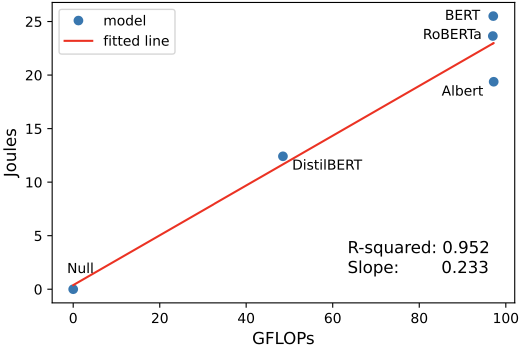Fig 5: Regression line on CPU energy consumption per step

Figure 6 shows an even stronger correlation with R² = 0.990. Both regression analyses have a p-value smaller than 0.01, making us reject the null hypothesis.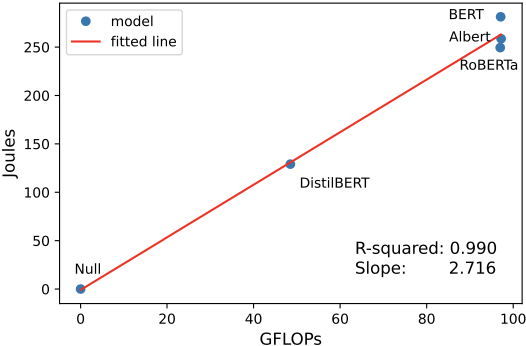Fig 6: Regression line on GPU energy consumption per step

Cold-Start

Measurements were taken on a deployed model with the web framework, Flask. Comparing the magnitude of the cold-start energy consumption to the other phases shows that the cold-start consumption is almost negligible. If the NLP model at hand needs substantial maintenance, the energy consumption will substantially impact the total consumption.

Inference

Figure 7 also shows that FLOPs are an excellent predictor of inference energy consumption. The strong correlation gives us the confidence to proceed with constructing the cost model.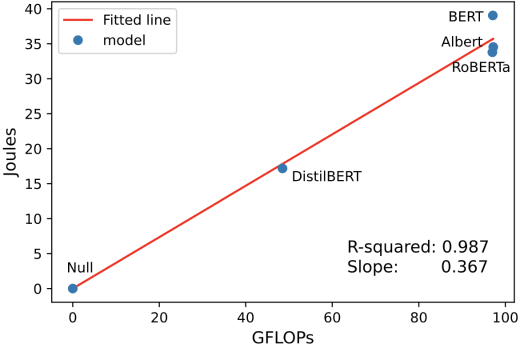Figure 7: Regression line on inference energy consumption per request

We now want to validate the study with new data points.

Scaling Up

With the test environment being able to accurately measure energy, we are now interested in whether our regression model can predict the energy consumption for a new model. The BERT Large model is a good option as it allows us to look at a larger model: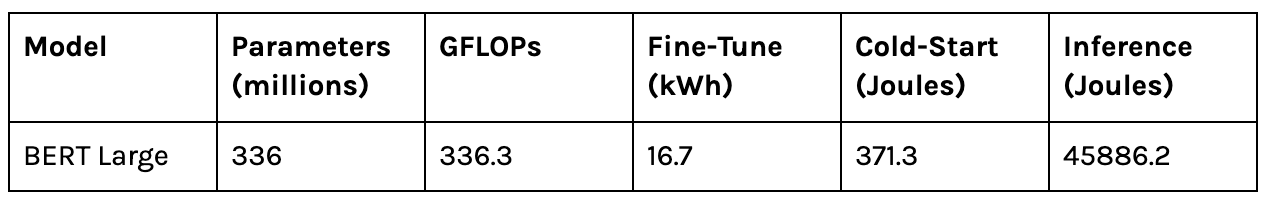Fig 8: Table of measured values of BERT Large

Now that we have the measured values, how well did they correlate with the predicted values from the regression model?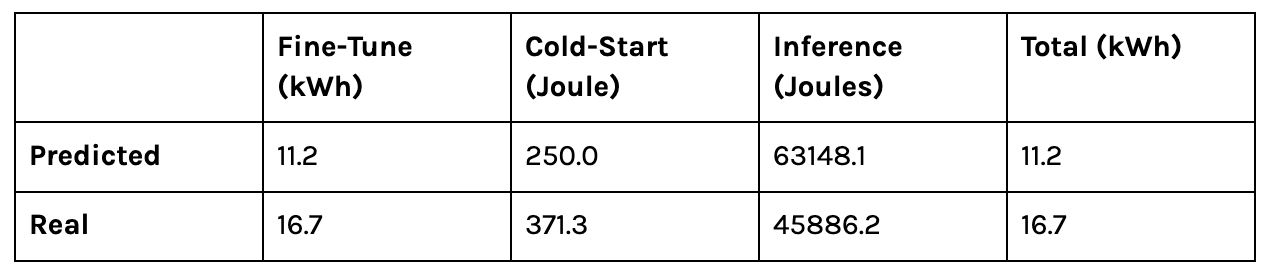Fig 9: Table of predicted energy consumption vs. real of BERT Large

The energy prediction underestimated the real total energy consumption by almost a third. How could it be so far off? If we examine the Fine-tuning phase, we see that the energy we did not expect came from the CPU.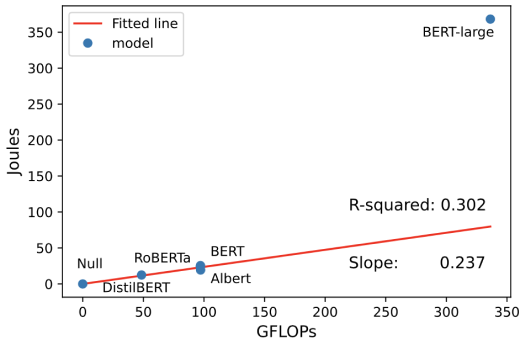Fig 10: Regression line on CPU energy consumption per step

Looking at Figure 10, we see that the R² value has decreased substantially. Let’s compare how much energy the memory used for the total CPU consumption and DRAM CPU. We find that memory used almost 22.0% of the energy when looking at the original data collected. For BERT Large, that percentage is only 15.8%. The CPU uses more energy than expected for the larger model.

Tokenizer

The choice of tokenizer also affects energy usage. In the Huggingface library, we can choose either the “Fast” implementation of the tokenizer or the basic one (Base).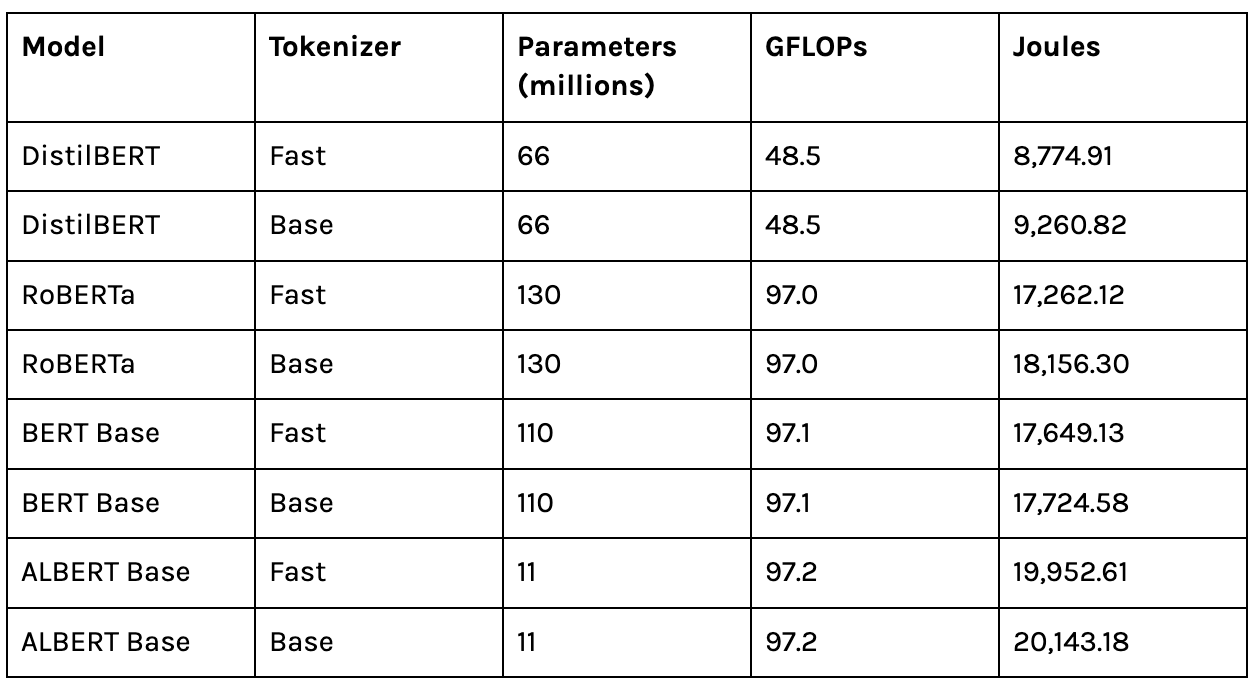Fig 11: Table of Models and Parameters, the work needed to execute the model, and the energy consumption for handling 1100 requests. Idle time energy consumption is excluded.

The measurements for the models above show that the fast tokenizer always results in lower energy consumption, even if the difference is small. If the entire AI community only used the fast tokenizer, the accumulated reduction in energy usage would have a significant impact on the environment.

Recent research presenting large transformer language models has shown promising results with high accuracy on the relationship between energy usage and the number of parameters. However, Table 4.3 shows that the number of parameters alone is not a great predictor of energy consumption.

Knowledge distillation, used to develop DistilBERT, is just one method to lower energy consumption. DistilBERT is around 50% more energy-efficient than other non-distilled models with similar accuracy.

## Conclusion: Just the Beginning

The lack of standardisation for measuring Machine Learning model size makes it difficult to derive an accurate cost model. With increased standardisation among tooling used in the AI community over the next few years, we will be able to more accurately predict energy usage when developing new models.

While this work has shown that FLOPs on their own are insufficient, the next step is finding other metrics to incorporate. Using model architecture-specific metrics such as layers, transformer units, and similar characteristics could be relevant factors to consider. Monitoring energy usage of models of various sizes would give us more data points and enable us to see how the correlation changes and whether the relationship is nonlinear.

With climate change becoming an even more pressing concern, and energy prices rising, reducing the energy consumption of our AI and ML models through smarter implementation choices will likely become much more common, providing economic and environmental benefits globally.

Datatonic are Google Cloud’s Machine Learning Partner of the Year with a wealth of experience developing and deploying impactful Machine Learning models and MLOps Platform builds. Need help with developing an ML model, or deploying your Machine Learning models fast? Have a look at our MLOps 101 webinar, where our experts talk you through how to get started with Machine Learning at scale, or get in touch to discuss your ML or MLOps requirements!

The Materials and Thesis that led to this article are available on Github.

Up next
Case Studies